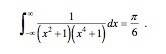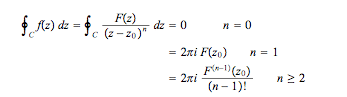# Contour integration

## Homework Statement

By an appropriate choice of contour, validate the following relationship by evaluation,## Homework Equations## The Attempt at a Solution

Any help on this one would be much appreciated.

#### Attachments

Last edited:

Cyosis
Homework Helper
I'm afraid copy pasting the relevant equations don't constitute as a attempt at a solution. What type of contour are you thinking of and do you know how to find the poles?

Edit: I believe the answer should be $\pi/2$

Last edited:
gabbagabbahey
Homework Helper
Gold Member
I'd start by finding all the singularities of the integrand...where are they? What type of singularities are they?

Put in z in place of the x's. The singularities are when the bottom line is zero, so theres a singularity at z = i and z = -i. The contour im thinking would be a semi circle on the positive side of the axis so -i isnt needed so z = i is one simple pole. I dont know how to handle the z^4 though.

Cyosis
Homework Helper
That is correct so far. You handle the z^4 term in the same manner, $(z^2+1)(z^4+1)=0$ gives you $(z^2+1)=0$ and $(z^4+1)=0$. Solve for z.

Ok, so i split ($$z^{2}$$ + 1) into (z+i)(z-i) to get the first pole. So am i right in spliting ($$z^{4}$$ + 1) into ($$z^{2}$$ + i)($$z^{2}$$ - i)? Do i then split each of those again?

Cyosis
Homework Helper
Don't need to split them further, just solve for z now.

Is z = $$\sqrt{i}$$ the other simple pole? I tried to do it out using that but i didnt get out $$\frac{\pi}{6}$$ as the final answer

Cyosis
Homework Helper
You should have a total of three poles within the contour, $\sqrt{i}$ is one of them.

As for not getting pi/6, read post #2.

Got it! Thanks a lot for all your help.